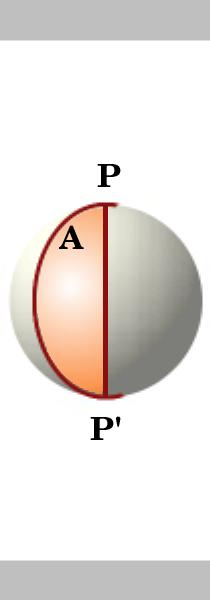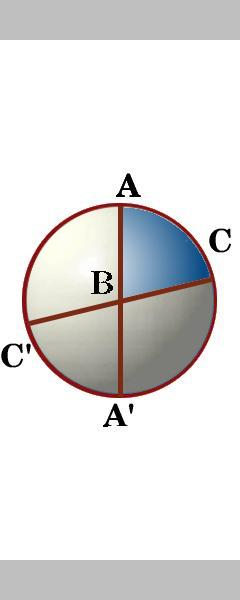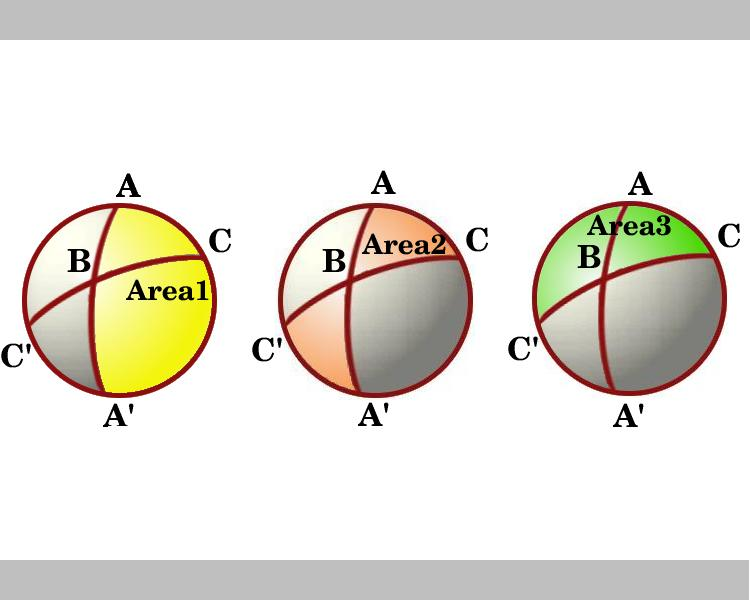# When the Angles of a Triangle Don't Add up to 180 Degrees

##### Age 14 to 18

Published May 2002,February 2011.

1. Introduction

Do the angles of a triangle add up to 180 degrees or $\pi$ radians? The answer is 'sometimes yes, sometimes no'. Is this an important question? Yes, because it leads to an understanding that there are different geometries based on different axioms or 'rules of the game of geometry'. Is it a meaningful question? Well no, at least not until we have agreed on the meaning of the words 'angle' and 'triangle', not until we know the rules of the game. In this article we briefly discuss the underlying axioms and give a simple proof that the sum of the angles of a triangle on the surface of a unit sphere is not equal to $\pi$ but to $\pi$ plus the area of the triangle. We shall use the fact that the area of the surface of a unit sphere is $4\pi$.

2. The Big Theorem

Before we can say what a triangle is we need to agree on what we mean by points and lines. We are working on spherical geometry (literally geometry on the surface of a sphere). In this geometry the space is the surface of the sphere; the points are points on that surface, and the line of shortest distance between two points is the great circle containing the two points. A great circle (like the Equator) cuts the sphere into two equal hemispheres. This geometry has obvious applications to distances between places and air-routes on the Earth.

Rotating sphere showing great circleThe angle between two great circles at a point P is the Euclidean angle between the directions of the circles (or strictly between the tangents to the circles at P). This presents no difficulty in navigation on the Earth because at any given point we think of the angle between two directions as if the Earth were flat at that point.

A lune is a part of the surface of the sphere bounded by two great circles which meet at antipodal points. We first consider the area of a lune and then introduce another great circle that splits the lune into triangles.

Rotating sphere showing 4 lunesLemma.

The area of a lune on a circle of unit radius is twice its angle, that is if the angle of the lune is A then its area is 2A. Two great circles intersecting at antipodal points P and P' divide the sphere into 4 lunes. The area of the surface of a unit sphere is $4\pi$.

The areas of the lunes are proportional to their angles at P so the area of a lune with angle A is

${\frac{A}{2\pi}\times {4\pi}= {2A}}$

Exercise 1.

What are the areas of the other 3 lunes? Do your 4 areas add up to $4\pi$?

The sides of a triangle ABC are segments of three great circles which actually cut the surface of the sphere into eight spherical triangles. Between the two great circles through the point A there are four angles. We label the angle inside triangle ABC as angle A, and similarly the other angles of triangle ABC as angle B and angle C.

Rotating sphere showing 8 trianglesExercise 2

Rotating the sphere can you name the eight triangles and say whether any of them have the same area? Check your answers here .

Theorem.

Consider a spherical triangle ABC on the unit sphere with angles A, B and C. Then the area of triangle ABC is

A + B + C - $\pi$.

The diagram shows a view looking down on the hemisphere which has the line through AC as its boundary. The regions marked Area 1 and Area 3 are lunes with angles A and C respectively. Consider the lunes through B and B'. Triangle ABC is congruent to triangle A'B'C' so the bow-tie shaped shaded area, marked Area 2, which is the sum of the areas of the triangles ABC and A'BC', is equal to the area of the lune with angle B, that is equal to 2B.

.So in the diagram we see the areas of three lunes and, using the lemma, these are:

Area 1 = 2A
Area 2 = 2B
Area 3 = 2C

In adding up these three areas we include the area of the triangle ABC three times. Hence

 Area 1 + Area 2 + Area 3 = Area of hemisphere +2(Area of triangle ABC) 2A + 2B + 2C = 2 $\pi$ + 2(Area of triangle ABC) Area of triangle ABC = A + B + C - $\pi$ .

3. Non-Euclidean Geometry

Sometimes revolutionary discoveries are nothing more than actually seeing what has been under our noses all the time. This was the case over the discovery of Non-Euclidean Geometry in the nineteenth century. For some 2000 years after Euclid wrote his 'Elements' in 325 BC people tried to prove the parallel postulate as a theorem in the geometry from the other axioms but always failed and that is a long story. Meanwhile mathematicians were using spherical geometry all the time, a geometry which obeys the other axioms of Euclidean Geometry and contains many of the same theorems, but in which the parallel postulate does not hold. All along they had an example of a Non-Euclidean Geometry under their noses.

Think of a line L and a point P not on L. The big question is: "How many lines can be drawn through P parallel to L?" In Euclidean Geometry the answer is exactly one" and this is one version of the parallel postulate. If the sum of the angles of every triangle in the geometry is $\pi$ radians then the parallel postulate holds and vice versa, the two properties are equivalent.

In spherical geometry, the basic axioms which we assume (the rules of the game) are different from Euclidean Geometry - this is a Non-Euclidean Geometry. We have seen that in spherical geometry the angles of triangles do not always add up to $\pi$ radians so we would not expect the parallel postulate to hold. In spherical geometry, the straight lines (lines of shortest distance or geodesics) are great circles and every line in the geometry cuts every other line in two points. The answer to the big question about parallels isIf we have a line L and a point P not on L then there are no lines through P parallel to the line L."

The Greek mathematicians (for example Ptolemy c 150) computed the measurements of right angled spherical triangles and worked with formulae of spherical trigonometry and Arab mathematicians (for example Jabir ibn Aflah c 1125 and Nasir ed-din c 1250) extended the work even further. The formula discussed in this article was discovered by Harriot in 1603 and published by Girard in 1629. Further ideas of the subject were developed by Saccerhi (1667 - 1733).

All this went largely un-noticed by the 19th century discoverers of hyperbolic geometry, which is another Non-Euclidean Geometry where the parallel postulate does not hold. In spherical geometry (also called elliptic geometry) the angles of triangles add up to more than $\pi$ radians and in hyperbolic geometry the angles of triangles add up to less than $\pi$ radians.

For further reading see the article by Alan Beardon 'How many Geometries Are There?' and the article by Keith Carne 'Strange Geometries' . There are some practical activities that you can try for yourself to explore these geometries further to be found at http://nrich.maths.org/MOTIVATE/conf8/index.html